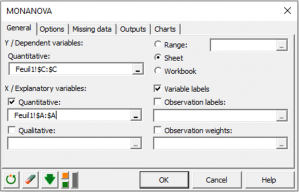# MONANOVA - Regresión Monotónica

MONANOVA and monotone regression involve a monotonic transformation of the dependent variables. They are available in Excel using the XLSTAT software.## The MONANOVA model definition

Monotone regression and the MONANOVA model differ only in the fact that the explanatory variables are either quantitative or qualitative. These methods are based on iterative algorithms based on the ALS (alternating least squares) algorithm. Their principle is simple, it consists of alternating between a conventional estimation using linear regression or ANOVA and a monotonic transformation of the dependent variables (after searching for optimal scaling transformations).

The MONANOVA algorithm was introduced by Kruskal (1965) and the monotone regression and the works on the ALS algorithm are due to Young et al. (1976).

These methods are commonly used as part of the full profile conjoint analysis. XLSTAT-Conjoint allows applying them within a conjoint analysis (see chapter on conjoint analysis based on full profiles) as well as independently.

The monotone regression tool (MONANOVA) combines a monotonic transformation of the responses to a linear regression as a way to improve the linear regression results. It is well suited to ordinal dependent variables.

XLSTAT-Conjoint analysis software allows you to add interactions and to vary the constraints on the variables.

## MONANOVA method

Monotone regression combines two stages: an ordinary linear regression between the explanatory variables and the response variable and a transformation step of the response variables to maximize the quality of prediction.

The algorithm is:

Run an OLS regression between the response variable Y and the explanatory variables X. We obtain the beta coefficients. Calculation of the predicted values of Y: Pred (Y) = beta * X Transformation of Y using a monotonic transformation (Kruskal, 1965) so that Pred(Y) and Y are close (using optimal scaling methods). Run an OLS Regression between Ytrans and the explanatory variables X. Thisgives new values for the beta. Steps 2 through 4 are repeated until the change in R² from one stage to another is smaller than the convergence criterion.### analice sus datos con xlstat

prueba gratuita de 14 días

Incluido en